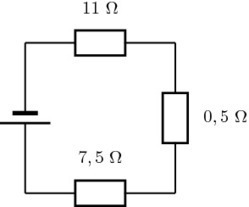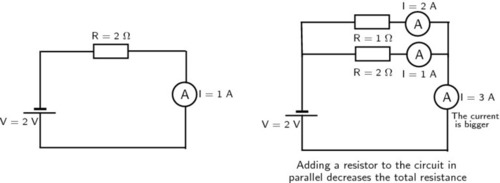Series resistorsChipa Thomas Maimela‘s insight:

When we add resistors in series to a circuit:

There is only one path for current to flow which ensures that the current is the same at every point in the circuit.

The voltage is divided across the resistors. The voltage across the battery in the circuit is equal to the sum of voltages across the series resistors:

See on everythingscience.co.za

Parallel ResistorsChipa Thomas Maimela‘s insight:

When we add resistors in parallel to a circuit:

There are more paths for current to flow which ensures that the current splits across the different paths.

The voltage is the same across the resistors. The voltage across the battery in the circuit is equal to the voltage across each of the parallel resistors:

See on everythingscience.co.za

Resolving forces into components

the angle between the horizontal and the incline is also the angle between the gravitational force and its component perpendicular to the inclined plane (this is normal to the plane). Using this angle and the fact that the components form part of a right-angled triangle we can calculate the components using trigonometry:

Chipa Thomas Maimela‘s insight:

Using trigonometry the components are given by:

Fgx=Fgsin(θ)Fgy=Fgcos(θ)

See on everythingscience.co.za

ACTIVITY

A ball bounces vertically on a hard surface after being thrown vertically up into the air by a boy standing on the ledge of a building.

Just before the ball hits the ground for the first time, it has a velocity of magnitude 15 m·s−1. Immediately, after bouncing, it has a velocity of magnitude 10 m·s−1.

The graph below shows the velocity of the ball as a function of time from the moment it is thrown upwards into the air until it reaches its maximum height after bouncing once.

Chipa Thomas Maimela‘s insight:

At what velocity does the boy throw the ball into the air?

What can be determined by calculating the gradient of the graph during the first two seconds?

Determine the gradient of the graph over the first two seconds. State its units.

How far below the boy’s hand does the ball hit the ground?

Use an equation of motion to calculate how long it takes, from the time the ball was thrown, for the ball to reach its maximum height after bouncing.

What is the position of the ball, measured from the boy’s hand, when it reaches its maximum height after bouncing?

See on everythingscience.co.za

Conservation of momentum in two dimensions

Pbefore=Pafter

Chipa Thomas Maimela‘s insight:

Example 1: 2D conservation of momentumQuestion

In a rugby game, Player 1 is running with the ball at 5 m·s−1 straight down the field parallel to the edge of the field. Player 2 runs at 6 m·s−1 an angle of 60° to the edge of the field and tackles Player 1. In the tackle, Player 2 stops completely while Player 1 bounces off Player 2. Calculate the velocity (magnitude and direction) at which Player 1 bounces off Player 2. Both the players have a mass of 90 kg.

See on everythingscience.co.za

Physical Sciences Break 1.0

Physical Sciences Grade 10-11-12.

Latest Stories from ScienceInsider

Physical Sciences Grade 10-11-12.

Science: This Week's News

Physical Sciences Grade 10-11-12.

The Davinci Institute of Technology

Research . Design . Education

Science current issue

Physical Sciences Grade 10-11-12.

Science Magazine Podcast

Physical Sciences Grade 10-11-12.